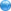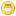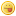- Inscription Langue :,,, … | Allez on remonte !
« Retourner à la documentation

## My CheckIO solutions

SPOILER ALERT: Those code aren't supposed to be viewable by people who haven't solved those problems yet themselves. If you wish to solve those by your own too, stay away: Spoilers ahead.

That also means that, yeah, some of my code might not be super beautiful. I didn't cheat, you are allowed to see others' solutions only after you have solved it yourself. Then sometimes you realize that some people did way more clever/beautiful than you.

In case I don't update this topic, there is the list of my solutions for users who are able to see them: http://www.checkio.org/user/JeromeJ/solutions/

All use Python 3.

Speech Module: http://www.checkio.org/mission/speechmodule/

Afficher le spoilerCacher le spoiler
Input: A number, an integer.

Output: A string representation of this number.

Example:

`checkio(4)=='four'checkio(143)=='one hundred forty three'checkio(12)=='twelve'checkio(101)=='one hundred one'checkio(212)=='two hundred twelve'checkio(40)=='forty'`

How it is used: This concept may be useful for the speech synthesis software or automatic reports systems. This system can also be used when writing a chatbot by assigning words or phrases numerical values and having a system retrieve responses based on those values.

Precondition: 0 < number < 1000

My solutions:

Second of JeromeJ for Speech Module http://www.checkio.org/mission/speechmodule/publications/JeromeJ/python-3/improved-one-line-poc-proof-of-concept/ (May 18, 2013)

`def checkio(number):    # I used the technique described here http://stackoverflow.com/a/8703135/1524913 to make a recursive lambda call     # Because I like one-line version (as a proof of concept)    return (lambda f: lambda n: f(f, n))(lambda f, n: next(v(n) for k,v in {range(0, 20): lambda r: (('', 'one', 'two', 'three', 'four', 'five', 'six', 'seven', 'eight', 'nine', 'ten', 'eleven', 'twelve', 'thirteen', 'fourteen', 'fifteen', 'sixteen', 'seventeen', 'eighteen', 'nineteen')[r]), range(20, 100): lambda r: ('', '', 'twenty', 'thirty', 'forty', 'fifty', 'sixty', 'seventy', 'eighty', 'ninety')[int(r/10)]+' '+f(f, int(str(n)[-1])), range(100, 1000): lambda r: f(f, int(r/100))+' hundred '+f(f, int(str(r)[-2:]))}.items() if n in k))(int(number)).strip() or 'zero'                 # Version with explanations. I didn't commented it so that syntax coloring is still effective     return (lambda f: # Function which will be called        lambda n: f(f, n))( # To make it recursive we have to call the function giving itself as first arg            lambda f, n: # The actual function. Note that it has to take itself as first argument                next(v(n) for k,v in {                    range(0, 20): lambda r: (('', 'one', 'two', 'three', 'four', 'five', 'six', 'seven', 'eight', 'nine', 'ten', 'eleven', 'twelve', 'thirteen', 'fourteen', 'fifteen', 'sixteen', 'seventeen', 'eighteen', 'nineteen')[r]),                    range(20, 100): lambda r: ('', '', 'twenty', 'thirty', 'forty', 'fifty', 'sixty', 'seventy', 'eighty', 'ninety')[int(r/10)]+' '+f(f, int(str(n)[-1])),                    range(100, 1000): lambda r: f(f, int(r/100))+' hundred '+f(f, int(str(r)[-2:]))                }.items() if n in k)    )(int(number)).strip() or 'zero' if __name__ == '__main__':    assert checkio(4) == 'four', "First"    assert checkio(133) == 'one hundred thirty three', "Second"    assert checkio(12)=='twelve', "Third"    assert checkio(101)=='one hundred one', "Fifth"    assert checkio(212)=='two hundred twelve', "Sixth"    assert checkio(40)=='forty', "Seventh, forty - it is correct"     print('All ok')`

First of JeromeJ for Speech Module: http://www.checkio.org/mission/speechmodule/publications/JeromeJ/python-3/one-line-poc-proof-of-concept/ (Nov. 8, 2012)

`def checkio(number):    # I wanted to take a special approach approach with this function and make a POC of the less ligne as possible     # Turning them into dict so that we can use its .get() method and never have some IndexError    spe = dict(enumerate(('', 'one', 'two', 'three', 'four', 'five', 'six', 'seven', 'eight', 'nine', 'ten', 'eleven', 'twelve', 'thirteen', 'fourteen', 'fifteen', 'sixteen', 'seventeen', 'eighteen', 'nineteen')))    decades = dict(enumerate(('', '', 'twenty', 'thirty', 'forty', 'fifty', 'sixty', 'seventy', 'eighty', 'ninety')))     # [::-1] so that the key for the unit is always 0    # [-2:] ignore the hundred if necessary    return (lambda r: r if r else 'zero')(' '.join(filter(lambda p: p, [(spe.get(d.get(2)), 'hundred'*bool(d.get(2)), decades.get(d.get(1)), spe.get(d.get(0)+10*(10<=int(str(number)[-2:])<20))) for d in (dict(enumerate(map(int, str(number)[::-1]))),)])))     # Note: spe and decades could also have been included in the one line but I prefer it this way. Note2: I'm sure this could be shortened againif __name__ == '__main__':    assert checkio(4) == 'four', "First"    assert checkio(133) == 'one hundred thirty three', "Second"    assert checkio(12)=='twelve', "Third"    assert checkio(101)=='one hundred one', "Fifth"    assert checkio(212)=='two hundred twelve', "Sixth"    assert checkio(40)=='forty', "Seventh, forty - it is correct"     print('All ok')`

Median: http://www.checkio.org/mission/median/

Afficher le spoilerCacher le spoiler
Input: A list of integers.

Output: The median, a float.

Example:

`checkio([1, 2, 3, 4, 5]) == 3checkio([3, 1, 2, 5, 3]) == 3checkio([1, 300, 2, 200, 1]) == 2checkio([3, 6, 20, 99, 10, 15]) == 12.5`

How it is used: The median has usage for Statistics and Probability theory, it has especially significant value for skewed distribution. For example: we want to know average wealth of people from a set of data -- 100 people earn \$100 in month and 10 people earn \$1,000,000. If we average it out, we get \$91,000. This is weird value and does nothing to show us the real picture. In this case the median would give to us more useful value and a better picture. The Article at Wikipedia.

Precondition:
1 < |X| ≤ 1000
∀ x ∈ X : 0 ≤ x < 10^6

My solution: http://www.checkio.org/mission/median/publications/JeromeJ/python-3/one-line-poc/ (Oct. 19, 2013)

`import math def checkio(data):    return (sorted(data)[int((len(data)-1)/2)]+sorted(data)[math.ceil((len(data)-1)/2)])/2 if __name__ == '__main__':    assert checkio([1, 2, 3, 4, 5]) == 3, "Sorted list"    assert checkio([3, 1, 2, 5, 3]) == 3, "Not sorted list"    assert checkio([1, 300, 2, 200, 1]) == 2, "It's not an average"    assert checkio([3, 6, 20, 99, 10, 15]) == 12.5, "Even length"`

Cypher Map(Old): http://www.checkio.org/mission/cipher-map/

Afficher le spoilerCacher le spoiler
Input: A list of two lists. The first list with four lines contain the Robot's cipher grille. The next list with four lines contain the square with the ciphered password. All the symbols in the square are lowercase Latin letters.

Example:

`checkio([['X...','..X.','X..X','....'],['itdf','gdce','aton','qrdi']]) == 'icantforgetiddqd' checkio([['....','X..X','.X..','...X'],['xhwc','rsqx','xqzz','fyzr']]) == 'rxqrwsfzxqxzhczy'`

How it is used: It will help you to learn how to work with 2D arrays.

My solution: http://www.checkio.org/mission/cipher-map/publications/JeromeJ/python-3/first/ (Feb. 13, 2013)

`class CypherMap:    def __init__(self, cypher_map):        self.map = cypher_map     def rotate(self):        r = self.map         self.map = [[cypher_line[i] for cypher_line in self.map][::-1] for i in range(len(self.map))]         return r     def getAllParsedMaps(self):        return [CypherMap.parse(self.rotate()) for i in range(4)]     @staticmethod    def parse(cypher_map):        return [[index for index, value in enumerate(cypher_line) if value == 'X'] for cypher_line in cypher_map]     @staticmethod    def r_join(piece):        return (piece if isinstance(piece, str) else "".join(map(CypherMap.r_join, piece))) def checkio(input_data):    """ Return password of given cipher map """     # I could have done it betterI may give it one more shot later!     cypher_map, cypher_pswd = input_data     return CypherMap.r_join(((cypher_pswd[i][index] for index in cypher_line) for i, cypher_line in enumerate(cypher_map) if cypher_line) for cypher_map in CypherMap(cypher_map).getAllParsedMaps()) if __name__ == '__main__':    assert checkio([[    'X...',    '..X.',    'X..X',    '....'],[    'itdf',    'gdce',    'aton',    'qrdi']]) == 'icantforgetiddqd', 'First'     assert checkio( [[    '....',    'X..X',    '.X..',    '...X'],[    'xhwc',    'rsqx',    'xqzz',    'fyzr']]) == 'rxqrwsfzxqxzhczy', 'Second'    print('All ok')`

Spaceship Purchase: http://www.checkio.org/mission/spaceship-purchase/

Afficher le spoilerCacher le spoiler
Input data: A list, that contains four integer numbers: Sofia's initial offer, Sofia's raise to her offer, the initial counteroffer from the old man, and the old man's reduction in his offer;
Output data: The amount of money that Sofia will pay for the spaceship. An integer.
Example:

`checkio([150, 50, 1000, 100]) == 450#Sofia: 200#Oldman: 900#Sofia: 250#Oldman: 800#Sofia: 300#Oldman: 700#Sofia: 350#Oldman: 600#Sofia: 400#Oldman: 500#Sofia: 450#... old man will be ok with it, because his next proposition will be lower than 450. # a bit shorter examplecheckio([500, 300, 700, 100]) == 700#Sofia will be ok with 700 because her next proposition will be higher`

My solution: http://www.checkio.org/mission/spaceship-purchase/publications/JeromeJ/python-3/first/ (Nov. 8, 2012)

`def checkio(offers):    '''       the amount of money that Petr will pay for the ride    '''    initial_petr, raise_petr, initial_driver, reduction_driver = offers    petr_price, driver_price = initial_petr, initial_driver     while True:        petr_price += raise_petr        if driver_price - reduction_driver > petr_price:            driver_price -= reduction_driver        else:            break     return (petr_price if petr_price < driver_price else driver_price)  if __name__ == '__main__':    assert checkio([150, 50, 1000, 100]) == 450, 'First'    assert checkio([150, 50, 900, 100]) == 400, 'Second'    print('All is ok')`

Afficher le spoilerCacher le spoiler
Input data: A string that is a password (Unicode for python 2.7).

Output data: The output will be true if the password is safe, a boolean or any data type that can be converted and processed as a boolean. In the results you will see the converted results.

Example:

`checkio('A1213pokl') == Falsecheckio('bAse730onE') == Truecheckio('asasasasasasasaas') == Falsecheckio('QWERTYqwerty') == Falsecheckio('123456123456') == Falsecheckio('QwErTy911poqqqq') == True`

How it is used: If you are worried about the security of your app or service, you can check your users' passwords for complexity. You can use these skills to require that your users passwords meet more conditions (punctuations or unicode).

Precondition:
The password contains only ASCII letters or digits.
`import re def checkio(data):    'Return True if password strong and False if not'    return bool(len(data) >= 10 and re.search('[0-9]', data) and re.search('[a-z]', data) and re.search('[A-Z]', data)) if __name__ == '__main__':    assert checkio('A1213pokl')==False, 'First'    assert checkio('bAse730onE4')==True, 'Second'    assert checkio('asasasasasasasaas')==False, 'Third'    assert checkio('QWERTYqwerty')==False, 'Fourth'    assert checkio('123456123456')==False, 'Fifth'    assert checkio('QwErTy911poqqqq')==True, 'Sixth'    print('All ok')`